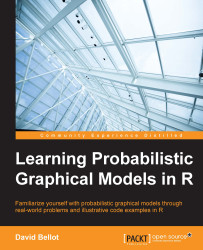•#### Learning Probabilistic Graphical Models in R#### Overview of this book

Probabilistic graphical models (PGM, also known as graphical models) are a marriage between probability theory and graph theory. Generally, PGMs use a graph-based representation. Two branches of graphical representations of distributions are commonly used, namely Bayesian networks and Markov networks. R has many packages to implement graphical models. We’ll start by showing you how to transform a classical statistical model into a modern PGM and then look at how to do exact inference in graphical models. Proceeding, we’ll introduce you to many modern R packages that will help you to perform inference on the models. We will then run a Bayesian linear regression and you’ll see the advantage of going probabilistic when you want to do prediction. Next, you’ll master using R packages and implementing its techniques. Finally, you’ll be presented with machine learning applications that have a direct impact in many fields. Here, we’ll cover clustering and the discovery of hidden information in big data, as well as two important methods, PCA and ICA, to reduce the size of big problems.
Learning Probabilistic Graphical Models in RCreditswww.PacktPub.comPrefaceFree Chapter
Probabilistic ReasoningExact InferenceLearning ParametersBayesian Modeling – Basic ModelsApproximate InferenceBayesian Modeling – Linear ModelsProbabilistic Mixture ModelsAppendixIndex## Summary

In this chapter we used the simple yet powerful Bayesian model, which has a representation as a probabilistic graphical model. We saw a Bayesian treatment of the over-fitting problem with the use of priors, such as the Dirichlet-multinomial and the famous Beta-Binomial model.

The last section introduced another graphical model, which was around before the invention of probabilistic graphical models and is called the Gaussian mixture. It is a very important model to capture data coming from different subsets within the same model. And finally, we saw another application of the EM algorithm: learning such models and finding out the parameters of each Gaussian component.

Of course, the Gaussian mixture is not the only latent variable model; in fact it represents a lot of Bayesian models and the probabilistic graphical model framework.

In the next chapter, we will continue our study of inference algorithms for Bayesian models and probabilistic graphical models with the introduction of a...